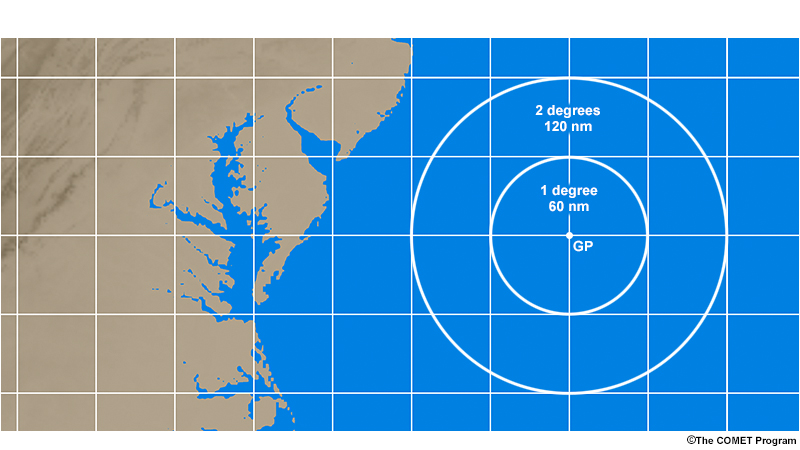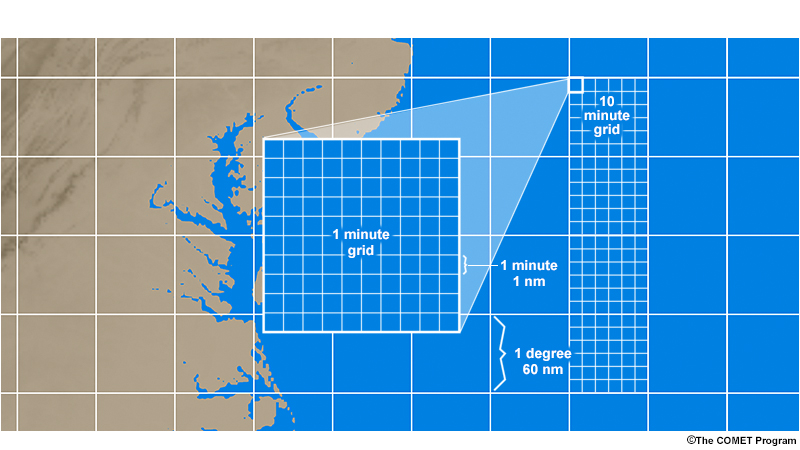Principles of Celestial Navigation

Basics » Degrees, Minutes, and Miles

But what does "being one degree away from its geographic position" really mean in terms of miles? On Earth, one degree of latitude equals 60 nautical miles. (A nautical mile is just a little bit larger than a normal, "statute" mile: 1 nautical mile (nm) = 1.15 miles). So if we measure a star's zenith distance, and it is one degree, then we are 60 nautical miles from its geographic position. If the zenith distance is two degrees, we are 120 nautical miles from its geographic position. And so on.In navigation, angular measurements—such as zenith distance—are typically not whole degrees, but also fractions of a degree. Similar to how an hour is made up of 60 minutes of time, each degree is made up of 60 minutes of arc, otherwise known as arcminutes. Since we just learned that on Earth one degree of latitude equals 60 nautical miles, then one minute of arc equals one nautical mile. An easy way to remember this is the saying "a mile a minute."This is an important point to remember: each minute of arc equals one mile (that is, "a mile a minute"), and each degree equals 60 miles.